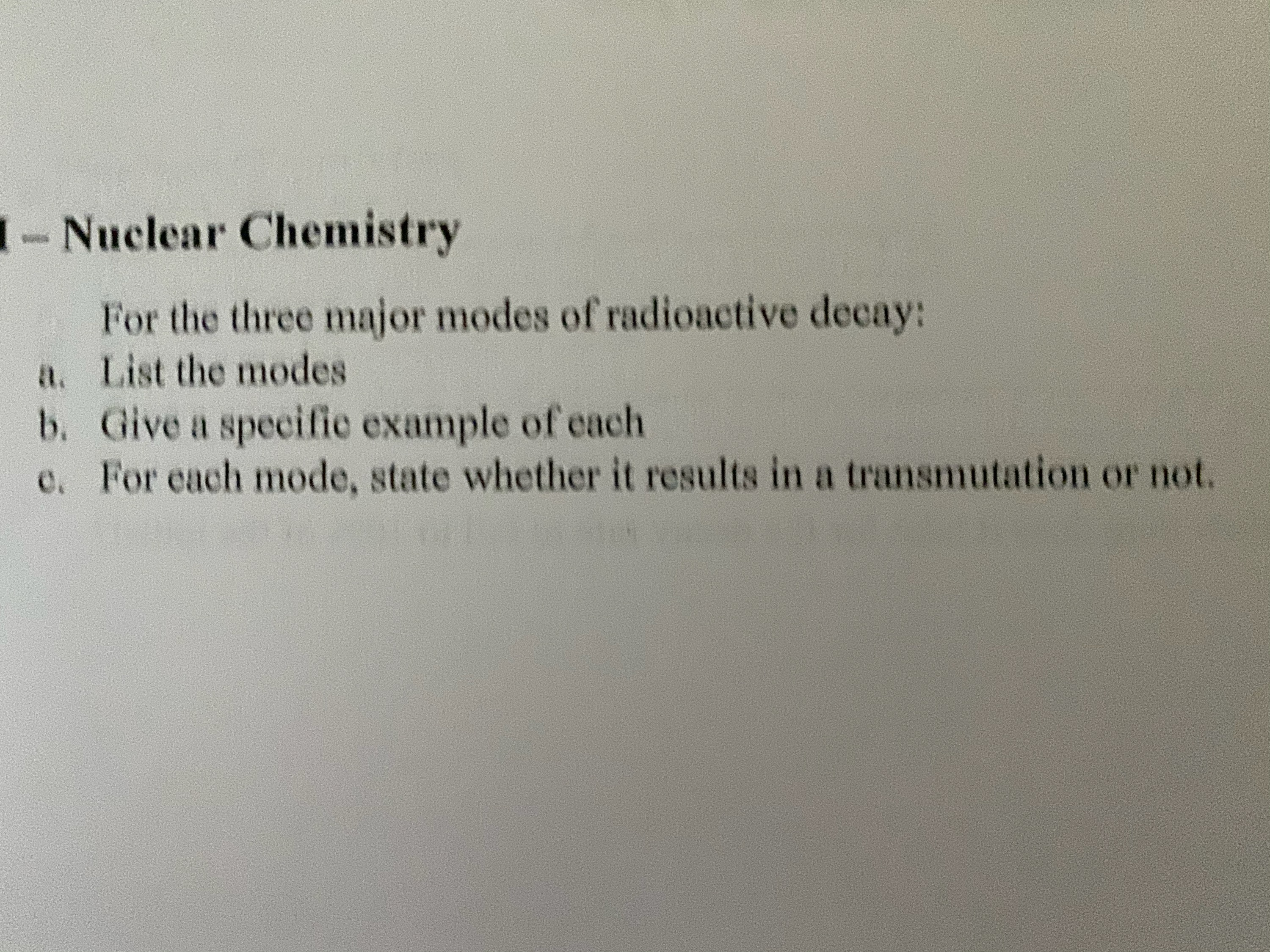# 1- Nuclear ChemistryFor the three major modes of radioactive decay:a. List the modesb. Give a specific example of eache. For each mode, state whether it results in a transmutation or not.

Question
1 viewshelp_outlineImage Transcriptionclose1- Nuclear Chemistry For the three major modes of radioactive decay: a. List the modes b. Give a specific example of each e. For each mode, state whether it results in a transmutation or not. fullscreen
check_circle

Step 1

Part A

The three major modes of radioactive decay are alpha, beta and gamma decay.

Step 2

Part B

The examples for each decay are given as,

Alpha Decay:

The alpha decay occurs as per the following reaction and the alpha decay of Polonium can be expressed as,

Step 3

Beta Decay

The beta decay occurs as per the following reaction a...

### Want to see the full answer?

See Solution

#### Want to see this answer and more?

Solutions are written by subject experts who are available 24/7. Questions are typically answered within 1 hour.*

See Solution
*Response times may vary by subject and question.
Tagged in

### Other